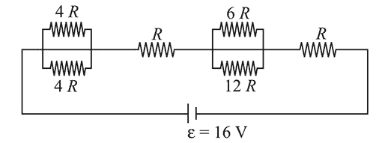# The resistive network shown below is connected to a D.C.Question:

The resistive network shown below is connected to a D.C. source of $16 \mathrm{~V}$. The power consumed by the network is 4 Watt. The value of $R$ is :1. (1) $6 \Omega$

2. (2) $8 \Omega$

3. (3) $1 \Omega$

4. (4) $16 \Omega$

Correct Option: , 2

Solution:

(2) Equivalent resistance,

$R_{\mathrm{eq}}=\frac{4 R \times 4 R}{4 R+4 R}+R+\frac{6 R \times 12 R}{6 R+12 R}+R$

$=2 R+R+4 R+R$

$=8 R$

Using, $P=\frac{V^{2}}{R_{\mathrm{eq}}}$

$4=\frac{16^{2}}{8 R}$

$\therefore R=\frac{16^{2}}{4 \times 8}=8 \Omega$